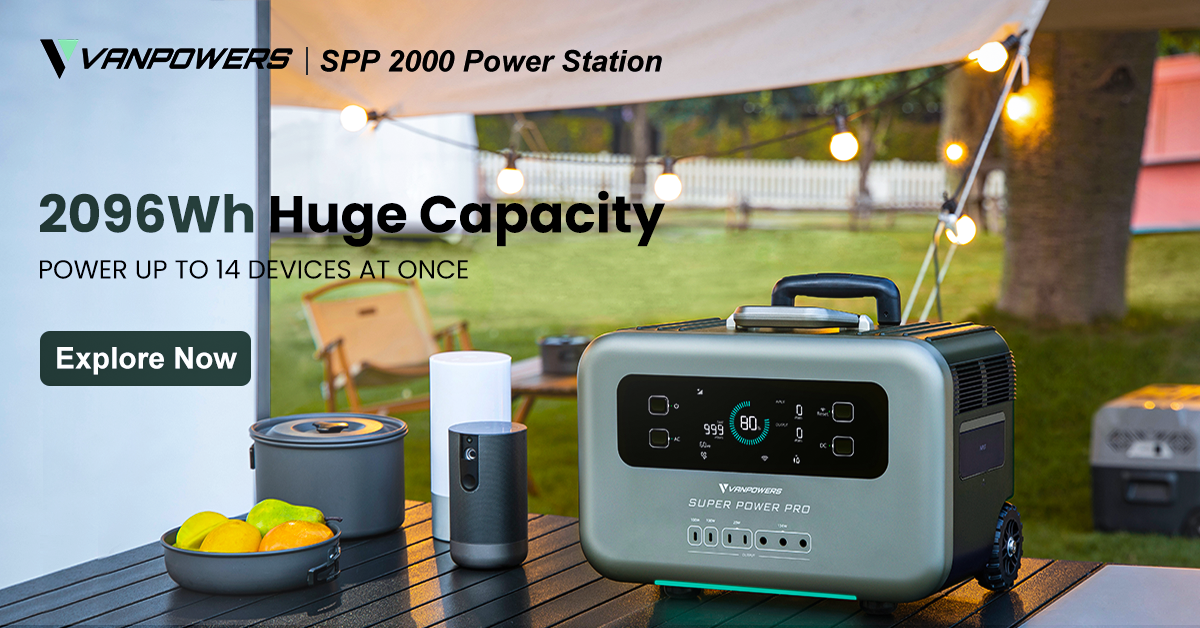## How to Calculate and Compare Watt Hours to Amp Hours

Nowadays, more and more types of power supply products are available on the market, such as power banks, portable power stations, and generators, that meet customers’ various power consumption needs in different situations. You will also find that the capacity of these products is listed in milliamp-hours or watt-hours. But when you want to compare the total amount of energy that different types of batteries can store, how do you come to the correct conclusion when they measure it in different units? We invite you to read the following guide to better understand what you see written on power station labels.

1. What is a watt-hour?

A watt-hour (Wh) is a unit of energy that measures the capacity of power (in watts) moving over time (an hour). When talking about battery life, this would mean the number of watts that an application uses per hour.

The equation is as follows:

(total number of watts) × (total number of hours) = watt-hours

For example, if you had a 60W bulb and you were trying to calculate how much energy your bulb uses in 2 hours, your equation would read:

60W × 2 hours = 120 watt-hours (120Wh)

1. What is an amp-hour?

An amp-hour is a measurement of current (in amps) flowing over a period of time (an hour). This measurement tells you the number of amps a device draws when you use it for an hour. It is expressed by the abbreviation “Ah.” One milliamp-hour (mAh) is equal to one thousandth of an amp-hour.

1. How do you convert mAh to Wh?

The equation is:

watt-hours = (milliamp-hours × volts) / 1000

For example, if you have a 300mAh 5V battery, you can multiply the milliamp hours by the volts and divide by 1000, like so:

(300mAh × 5V) / 1000 = 1.5Wh

1. How do you convert Wh to mAh?

The equation is:

milliamp-hours = (watt-hours × 1000) / volts

For example, if you have a 1.5Wh battery rated at 5V, the power is calculated as:

(1.5Wh × 1000) / 5V = 300mAh

1. Why are the capacities of our portable power stations listed in Wh instead of mAh?

Because milliamp-hours don’t take voltage into account, and because voltage affects the amount of power a battery can deliver, mAh ratings are imperfect across similar batteries and just plain bad for comparing different types of batteries. For example, a 12V 10Ah automotive battery has an actual capacity of 120Wh (12V × 10Ah = 120Wh), but a 10Ah laptop battery at 3.6 volts has a capacity of only 36Wh (3.6V × 10Ah = 36Wh). Even though the amp-hours are the same, the actual stored power is three times greater in the car battery. In short, comparing different types of batteries in amp-hours or milliamp-hours is like comparing apples to oranges; watt-hours make the comparison apples to apples. When you want to buy a portable power station, it’s important to understand the actual usable battery capacity in order to make informed choices, and watt-hours are a clearer measure.